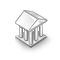Interesting script?
So post a link to it -
let others appraise it

You liked the script? Try it in the MetaTrader 5 terminalPNN Neural Network Class - library for MetaTrader 5

Views:
4815
Rating:
Published:
2012.12.25 11:31

The CNetPNN class realizes the probabilistic neural network (Probabilistic Neural Network - PNN).

Creation of the network is declared to be the class parametric constructor.

CNetPNN *net=new CNetPNN(input vector size, the number of classes (classification targets));

The numbering of classes (classification targets) starts from zero and must be continuous. For example, if 3 classes are set, the number of classes must be: 0, 1, 2.

Learning network is provided by calling the Learn method (the number of learning patterns, input data array, output data array, the number of learning cycles, the maximum learning error).

Input and output learning data are located in one-dimensional arrays vector by vector. On every input learning vector must be defined the class number in the input data. The process of learning is restricted either by epochs of learning, or allowable error.

The Learn method returns the following values:

•  0 - network learning is completed and learning result can be checked through the class variable: mse – learning error, epoch – number of accomplished learning cycles;
• -1 - non-existent class of the input learning data;
• -4 - not enough memory.

To get a response of the network serves the Calculate method (input vector array). The Calculate method returns the class number corresponding to input vector, or -1, if the network is not learned.

Save (open file handle with FILE_WRITE and FILE_BIN flags) and Load (open file handle with FILE_READ and FILE_BIN flags) methods are intended for saving the network to a file and loading the network from the file respectively. Network topology, learning errors and array weights are saved to the file. If parameters of the loaded network topology differ from the parameters of the established network topology, the network will not be loaded and the Load method will return false.

Using of the class is shown in the attached specimen: Test_PNN_XOR - learning network function "exclusive OR".

Translated from Russian by MetaQuotes Software Corp.
Original code: https://www.mql5.com/ru/code/1323ATR_MA_Oscillator

ATR MA Oscillator is the oscillator drawn on the basis of the difference between the ATR oscillator and its signal line.i-VaR95

The indicator displays the historical volatility valueGRNN Neural Network Class

The class realizes generalized regression network (General Regression Neural Network - GRNN)iSarX4

Four parabolic indicators at one time!